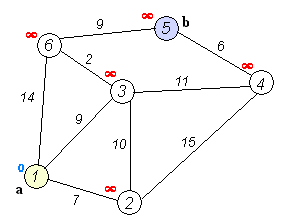Fig 1: This graph shows the shortest path from node “a” or “1” to node “b” or “5” using Dijkstras Algorithm. The visited nodes will be colored red. You will see the final answer (shortest path) is to traverse nodes 1,3,6,5 with a minimum cost of 20.

Dijkstra’s Algorithm is a graph search algorithm that solves the single-source shortest path problem for a graph with non-negative edge path costs, producing a shortest path tree. Concieved by Edsger Dijkstra.

# Condition:

• Both directed and undirected graphs
• All edges must have nonnegative weights
• Graph must be connected

# Algorithm:

Let the node at which we are starting be called the initial node. Let the distance of node Y be the distance from the initial node to Y. Dijkstra’s algorithm will assign some initial distance values and will try to improve them step by step.

1. Assign to every node a tentative distance value: set it to zero for our initial node and to infinity for all other nodes.
2. Mark all nodes unvisited. Set the initial node as current. Create a set of the unvisited nodes called the unvisited set consisting of all the nodes.
3. For the current node, consider all of its unvisited neighbors and calculate their tentative distances. For example, if the current node A is marked with a distance of 6, and the edge connecting it with a neighbor B has length 2, then the distance to B (through A) will be 6 + 2 = 8.
4. When we are done considering all of the neighbors of the current node, mark the current node as visited and remove it from the unvisited set. A visited node will never be checked again.
5. If the destination node has been marked visited (when planning a route between two specific nodes) or if the smallest tentative distance among the nodes in the unvisited set is infinity (when planning a complete traversal; occurs when there is no connection between the initial node and remaining unvisited nodes), then stop. The algorithm has finished.
6. Select the unvisited node that is marked with the smallest tentative distance, and set it as the new “current node” then go back to step 3.

# Edsger Dijkstra (1930–2002)

Edsger Dijkstra’s parents were Douwe Wybe Dijkstra and Brechtje Cornelia Kluijver (or Kluyver); he was the third of their four children. His father taught chemistry at the high school in Rotterdam while his mother was trained as a mathematician although she never had a formal position. Dijkstra wrote later of his mother’s mathematical influence on him “she had a great agility in manipulating formulae and a wonderful gift for finding very elegant solutions”.He published this shortest distance algorithm, together with his very efficient algorithm for the shortest spanning tree, were published in the two page paper A Note on Two Problems in Connexion with Graphs (1959). Also in 1959 he was awarded his Ph.D. from the University of Amsterdam for his thesis Communication with an Automatic Computer

# Worst Case Running Time Complexity

O(|E|+|V|log|V|)

Every time the main loop executes, one vertex is extracted from the queue. Assuming that there are V vertices in the graph, the queue may contain O(V) vertices. Each pop operation takes O(log V) time assuming the heap implementation of priority queues. So the total time required to execute the main loop itself is O(V log V). In addition, we must consider the time spent in the function expand, which applies the function handle_edge to each outgoing edge. Because expand is only called once per vertex, handle_edge is only called once per edge. It might call push(v’), but there can be at most V such calls during the entire execution, so the total cost of that case arm is at most O(V log V). The other case arm may be called O(E) times, however, and each call to increase_priority takes O(log V) time with the heap implementation. Therefore the total run time is O(V log V + E log V), which is O(E log V) because V is O(E) assuming a connected graph.

(There is another more complicated priority-queue implementation called a Fibonacci heap that implements increase_priority in O(1) time, so that the asymptotic complexity of Dijkstra’s algorithm becomes O(V log V + E); however, large constant factors make Fibonacci heaps impractical for most uses.)

If you want to read up on more graph problems or Discrete Math topics in general a great book to easily learn and practice these topics is Practice Problems in Discrete Mathematics by Bojana Obrenic’, and Discrete Math Workbook: Interactive Exercises by James R. bush.

Thanks for reading this article I hope its helpful to you all ! Keep up the learning, and if you would like more computer science, programming and algorithm analysis videos please visit and subscribe to my YouTube channels (randerson112358 & compsci112358 )

# Check Out the following for content / videos on Computer Science, Algorithm Analysis, Programming and Logic:

compsci112358:

Video Tutorials on Recurrence Relation:

Video Tutorial on Algorithm Analysis:
https://www.udemy.com/algorithm-analysis/

Written by